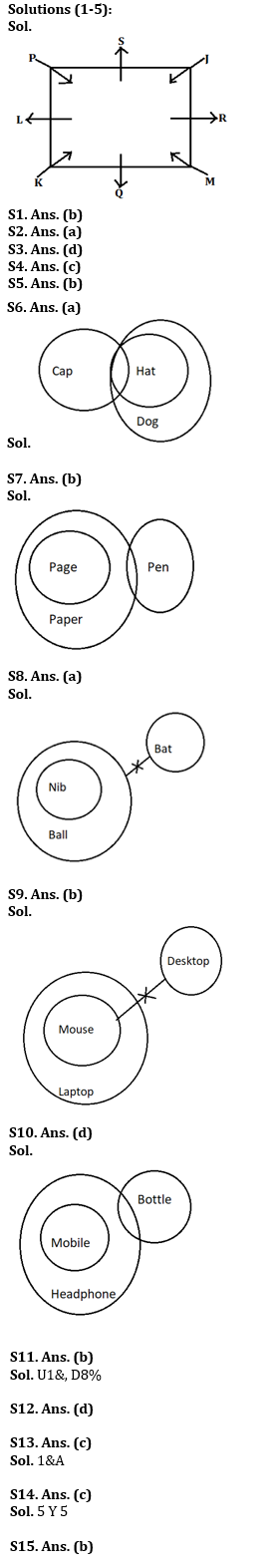Latest Banking jobs   »

# Reasoning Ability Quiz For IBPS RRB PO Clerk Prelims 2022- 6th August

Directions (1-5): Study the following information carefully and answer the questions given below:
Eight persons J, K, L, M, P, Q, R and S sit around the square table in such a way four of them sit at four corners and face the center while four of them sit in the middle of each of the four sides and face outside.
J faces the center and sits third to the left of Q. P faces the center and is not an immediate neighbour of Q. Only one person sits between Q and R. M sits second to right of K who faces the center. L is not an immediate neighbour of J.

Q1. Who among the following are immediate neighbours of S?
(a) R, M
(b) P, J
(c) P, L
(d) J, M
(e) K, M

Q2. What is the position of L with respect to P?
(a) Immediate right
(b) Second to the right
(c) Immediate left
(d) Third to the left
(e) None of these

Q3. Four of the following five belong to a group in a certain way, find which of the one does not belong to the group?
(a) S
(b) L
(c) R
(d) M
(e) Q

Q4. Who among the following person sits opposite to R?
(a) S
(b) M
(c) L
(d) K
(e) None of these

Q5. How many maximum persons can sit between P and M?
(a) 6
(b) 3
(c) 2
(d) 4
(e) 5

Directions (6-10): In each of the questions below, some statements are given followed by two conclusions. You have to take the given statements to be true even if they seem to be at variance with commonly known facts. Read all the conclusions and then decide which of the given conclusions logically follows from the given statements disregarding commonly known facts.
(a) If only conclusion I follows.
(b) If only conclusion II follows.
(c) If either conclusion I or II follows.
(d) If neither conclusion I nor II follows.
(e) If both conclusions I and II follow.

Q6. Statements:
Some Cap are Hat.
All Hat are Dog.
Conclusion
I: All Cap can be Dog.
II: All Dog are Hat

Q7. Statements:
Some Paper are Pen.
All Page are Paper.
Conclusion
I: Some Pen are Page
II: Some Pen can be Page

Q8. Statements:
Ball is Nib.
No Ball are Bat.
Conclusion
I: No Nib is Bat
II: Some Nib can be Bat

Q9. Statements:
Only Laptop are Mouse.
No Mouse are Desktop.
Conclusion
I: All Laptop can be Desktop
II: Some Laptop can be Desktop

Q10. Statements:
Conclusion
I: Some Mobile are Bottle

Directions (11-15): Study the following alphanumeric series carefully and answer the questions given below:
S * 7 B \$ * G 2 8 U 1 & A V # F 3 5 H @ 5 Y 5 M 3 2 D 8 % N 2 4

Q11. How many such numbers are there which is immediately preceded by alphabets and immediately followed by symbols?
(a) One
(b) Two
(c) Three
(d) Four
(e) None

Q12. Which of the following element is 19th from right end of the series?
(a) #
(b) F
(c) 3
(d) V
(e) None of these

Q13. How many such symbols are there which is immediately preceded by numbers and immediately followed by vowels?
(a) Two
(b) None
(c) One
(d) Four
(e) Three

Q14.How many such alphabets are there which is immediately preceded and followed by same number?
(a) Three
(b) Two
(c) One
(d) Four
(e) None

Q15. If all numbers are eliminated from the series, then, which of the following element is 11th from right end of the series?
(a) V
(b) A
(c) &
(d) #
(e) None of these

Solutions#### Congratulations!Union Budget 2023-24: Free PDF